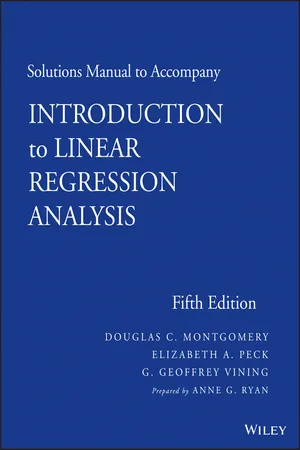eBook - ePub

# Solutions Manual to accompany Introduction to Linear Regression Analysis

## Douglas C. Montgomery, Elizabeth A. Peck, G. Geoffrey Vining

Share book
English
ePUB (mobile friendly)
Available on iOS & Android
eBook - ePub

# Solutions Manual to accompany Introduction to Linear Regression Analysis

## Douglas C. Montgomery, Elizabeth A. Peck, G. Geoffrey Vining

Book details
Book preview
Citations

As the Solutions Manual, this book is meant to accompany the main title, Introduction to Linear Regression Analysis, Fifth Edition.Clearly balancing theory with applications, this book describes both the conventional and less common uses of linear regression in the practical context of today's mathematical and scientific research. Beginning with a general introduction to regression modeling, including typical applications, the book then outlines a host of technical tools that form the linear regression analytical arsenal, including: basic inference procedures and introductory aspects of model adequacy checking; how transformations and weighted least squares can be used to resolve problems of model inadequacy; how to deal with influential observations; and polynomial regression models and their variations. The book also includes material on regression models with autocorrelated errors, bootstrapping regression estimates, classification and regression trees, and regression model validation.

How do I cancel my subscription?
Simply head over to the account section in settings and click on “Cancel Subscription” - it’s as simple as that. After you cancel, your membership will stay active for the remainder of the time you’ve paid for. Learn more here.
What is the difference between the pricing plans?
Both plans give you full access to the library and all of Perlego’s features. The only differences are the price and subscription period: With the annual plan you’ll save around 30% compared to 12 months on the monthly plan.
What is Perlego?
We are an online textbook subscription service, where you can get access to an entire online library for less than the price of a single book per month. With over 1 million books across 1000+ topics, we’ve got you covered! Learn more here.
Do you support text-to-speech?
Look out for the read-aloud symbol on your next book to see if you can listen to it. The read-aloud tool reads text aloud for you, highlighting the text as it is being read. You can pause it, speed it up and slow it down. Learn more here.
Is Solutions Manual to accompany Introduction to Linear Regression Analysis an online PDF/ePUB?
Yes, you can access Solutions Manual to accompany Introduction to Linear Regression Analysis by Douglas C. Montgomery, Elizabeth A. Peck, G. Geoffrey Vining in PDF and/or ePUB format, as well as other popular books in Mathématiques & Probabilités et statistiques. We have over one million books available in our catalogue for you to explore.

## Information

Publisher
Wiley
Year
2013
ISBN
9781118548509# Multiple Linear Regression

3.1 a.= −1.8 + .0036x2 + .194x7 − .0048x8
b. Regression is significant.c. All three are significant.
 Coefficient test statistic p-value β2 5.18 0.000 β7 2.20 0.038 β8 −3.77 0.001
d. R2 = 78.6% and R2Adj = 76.0%
e. F0 = (257.094 − 243.03)/2.911 = 4.84 which is significant at α = 0.05. The test statistic here is the square of the t-statistic in part c.
3.2 Correlation coefficient between yi andi is .887. So (.887)2 = .786 which is R2.
3.3 a. A 95% confidence interval on the slope parameter β7 is7 ± 2.064(.08823) = (.012, .376)
b. A 95%. confidence interval on the mean number of games won by a team when x2 = 2300, x7 = 56.0 and x8 = 2100 is3.4 a.= 17.9 + .048x7 − .00654x8 with F = 15.13 and p = 0.000 which is significant.
b. R2 = 54.8% and R2Adj = 51.5% which are much lower.
c. For β7, a 95% confidence interval is 0.484 ± 2.064(.1192) = (−.198, .294) and for the mean number of games won by a team when x7 = 56.0 and x8 = 2100, a 95% confidence interval is 6.926 ± 2.064(.533) = (5.829,8.024). Both lengths are greater than when x2 was included in the model.
d. It can affect many things including the estimates and standard errors of the coefficients and the value of R2.
3.5 a.= 32.9 − .053x1 + .959x6
b. Regression is significant.c. R2 = 78.6% and R2Adj = 77.3%. For the simple linear regression with x1, R2 = 77.2%.
d. A 95% confidence interval for the slope parameter β1 is −.053 ± 2.045(.006145) = (−.0656, −.0405).
e. x1 is significant while x6 is not.
 Coefficient test statistic p-value β1 -8.66 0.000 β6 1.43 0.163
f. A 95% confidence interval on the mean gasoline mileage when x1...